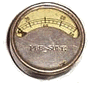# Tiny Tv

This page describes fixtures used by WardCunningham to test a microcontroller program based on data originally worked up in an Excel spreadsheet. It is one of several SampleApplications featured on this site.

Take a peek at the test results here,

or read on to see how they were acquired.TinyTv generating circles using the finite difference method.

Background

This project represents the convergence of three other projects that I have explored as part of my work as a hands-on methodologist.

• VideoCircles -- Realtime evaluation of a polynomial equation by finite difference methods can be realized as hardware or simple software that runs at video rates. This spreadsheet compares the finite difference  method to direct evaluation of the polynomial as an excel formula.

• TinyTv -- Software that generates NTSC video signals, such as test patterns or simple readouts, using programmed i/o in a low cost microcontroller  and a resistor network for the video dac.

• Codosome -- An object model of microcontroller hardware and software that can make the practices of ExtremeProgramming workable in a resource starved environment. Codosome includes a java simulator that can run partially written microcontroller programs.

In this project, I used the Fit framework to acceptance test a TinyTv program that generates a display of concentric circles using the finite difference method explored in VideoCircles. The Codosome simulator allows me to represent only the video generation part of the program (as opposed to the NTSC sync timing part of the program) and to test that against intermediate and final results obtained in the VideoCircles spreadsheet.

Fixtures

Two new fixtures were created corresponding to the two types of tables found in the VideoCircles spreadsheet.

• Coefficients -- This table contains the five input parameters to the computation corresponding to the coefficients (a through e) of the polynomial, ax^2 + by^2 + cx + dy + e.

• Values -- These tables contain intermediate or final results organized as an x-y grid that corresponds to the x-y raster that television uses to paint the screen. Each row corresponds to a horizontal scan line. Each cell of a row holds the value that a variable will take on at the corresponding moment of the scan.

Both fixtures interpret very specific positional formats that is best implemented by making refinements of PrimitiveFixture.

The spreadsheet rasters are much smallter than those that would be generated while producing a television image. The Values fixture interacts with the Codosome simulator to extract values of the same dimension as the tables it is given. It does so by forcing a reset with each doTable and a horizontal sync interrupt with each doRow.

Results

A word document was prepared describing the computation to be performed by the TinyTv implementation of VideoCircles. Tables were copied from the VideoCircles spreadsheet and edited to include the fixture names on the first line of each table.

You will notice that the test output shows that the Codosome implementation does not yet match the spreadsheet results. I stopped short of perfection in this case study so that you can see the sorts of test results I had to guide my programming. I ordered the tables in the test with the least dependent variables first. My strategy is to make each of these tables green in turn. I have two more tables to go.

References

Last edited October 13, 2003